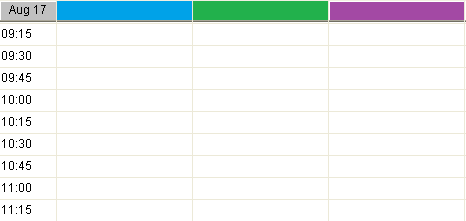Category: PowerBuilder
Hits: 1462

User Rating: 3 / 5Here is a way to build a grid datawindow which contains columns corresponding to an unknown number of data elements. You could use this approach in creating a project schedule, inventory location system, baseball box score, or any number of other examples. My example assumes the minimum number of columns to be four.

This create grid event returns a string.

long    ll_columns, ll_count, ll_pos
string ls_errors, ls_sql, ls_dw_presentation, ls_dw_syntax, ls_find, ls_name, ls_mod_string
// base presentation information
ls_dw_presentation =    "style( type=Grid                          &
datawindow( units=1                       &
Color= 16777215)              &
column( Font.Face='Arial'         &
Font.Height=-9                    &
Font.Weight=400)                  &
text(   Font.Face='Arial' &
Font.Height=-9                    &
Font.Weight=400                   &
Border=6)"

// set initial grid to 4 columns (plus time_of_day and last_col columns)
ls_sql = "SELECT space(10) as time_of_day, space(40) as col1, space(40) as col2, space(40) as col3, space(40) as col4,"

ll_count = 5 // next column to add would be 5

// this is the variable number of data elements
ll_columns = ids_trains.rowcount()

DO WHILE ll_count <= ll_columns
ls_sql += " space(40) as col" + string(ll_count) + ","
ll_count++
LOOP

ls_sql += " '' as last_col"
ls_sql += " FROM dbo.TRAINS WITH (NOLOCK) WHERE 1 = 2"
// build the datawindow syntax from the presentation and sql statement
ls_dw_syntax = SQLCA.Syntaxfromsql( ls_sql, ls_dw_presentation, ls_errors)
IF Len(ls_errors) = 0 THEN
// widen the header band a bit
// create dwo and assign to grid
dw_grid.create(ls_dw_syntax,ls_errors)
END IF
// appearance modifies
dw_grid.modify("last_col.visible=FALSE")

FOR ll_count = 1 TO Long(dw_grid.Object.DataWindow.Column.Count)
IF dw_grid.Describe("#" + String(ll_count) + ".Visible") = "1" Then
ls_name = dw_grid.Describe("#" + String(ll_count) + ".Name")
ls_mod_string = ls_name + "_t.background.mode=0" // must set to opaque or color does not show
ls_errors = dw_grid.modify(ls_mod_string)
// set the column heading color
ls_mod_string = ls_name + "_t.background.color=12632256"
ls_errors = dw_grid.modify(ls_mod_string)
IF POS(ls_name ,'time_of_day') = 0 THEN
ls_mod_string = ls_name + "_t.Alignment=0" //left
ls_errors = dw_grid.modify(ls_mod_string)
END IF
ls_mod_string = ls_name + "_t.Height=18"
ls_errors = dw_grid.modify(ls_mod_string)
ls_mod_string = ls_name + "_t.tooltip.enabled=1"
ls_errors = dw_grid.modify(ls_mod_string)
END IF
NEXT
RETURN ls_errors

Now I know you can do several modifies with a single statement but it is much easier to debug if you do a single attribute at a time.

Once you have the grid set up you can call subsequent methods to populate it (and make columns invisible if needed), resize the width of the columns, etc.Example grid for a time schedule with fifteen minute increments.

We use cookies which are necessary for the proper functioning of our websites. We also use cookies to analyze our traffic, improve your experience and provide social media features. If you continue to use this site, you consent to our use of cookies.